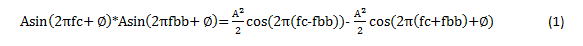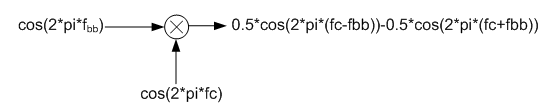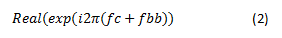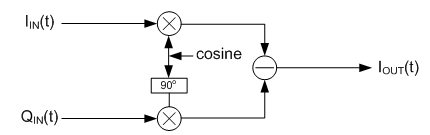# Digital signal processing in RF sampling DACs – part 2

In this blog post, I will describe mixers and numerically controlled oscillators (NCOs), which are the basic building blocks used to implement digital up converters (DUCs) in the RF sampling digital-to-analog converter (DAC) signal chain.

In the previous installment of this series, I explained the use of interpolation filters to increase the data rate of the input signal to more than twice the desired output RF signal frequency. If the final data rate after interpolation is much higher than twice the desired RF output frequency, you can easily filter out the image of the desired signal in the second Nyquist zone.

Mixers and NCOs perform the important function of moving a signal from one frequency location to another (also known as frequency translation) within the first Nyquist zone. Frequency translation occurs when a lower-frequency signal modulates a higher-frequency signal in the time domain. Common names used for the high and low frequency signals are the local oscillator (or carrier) frequency and the baseband signal frequency, respectively.

To understand how frequency translation works, consider the effect of multiplying two pure sinusoids with equal amplitude (A), equal phase (Φ) and frequencies at fc and fbb, as shown by Equation 1:where fc and fbb are the carrier frequency and baseband frequency, respectively.

Figure 1 illustrates a case where A = 1 and Φ = 0.Figure 1: Implementation of a real mixer

To simplify, I will only focus on a case where the desired RF signal frequency is higher than the carrier frequency, known as low-side injection. Low-side injection has an advantage in that the RF spectrum is not a mirrored image of the original baseband signal spectrum.

From Equation 1, the output of the multiplier has two signals with the same energy located at frequencies fc+fbb (this is the desired signal) and fc-fbb (this is an unwanted image). This multiplier also describes a real mixer since the carrier and baseband signals are both real valued. A significant drawback of real mixing is that both the desired signal and unwanted image have equal energy and are separated by twice the baseband signal frequency. It is not practical to filter out the unwanted image in a real mixer because the baseband signal frequency is usually low. Consequently, complex valued baseband and carrier signals when used in complex mixers produce an output at one frequency (fc+fbb) only. Equation 2 expresses the output of a complex mixer as:Complex mixing results in a perfect cancellation of the unwanted sideband image if the gain of the real (IIN(t)) and imaginary (QIN(t)) inputs to the mixer are perfectly matched and their phase offset is exactly 90 degrees. Any deviation from this requirement creates a sideband image at frequency fc-fbb, with energy proportional to the magnitude of the gain and phase imbalance. This requirement is difficult to achieve in an analog mixer where gain and phase can vary randomly with process, voltage and temperature (PVT) variations and limits the usefulness of analog complex mixers to high intermediate frequency (IF) heterodyne transmitters. However, RF sampling DACs like the DAC38RF83 use digital complex mixers for frequency translation and are able to achieve perfect cancellation of the sideband image independent of PVT variations. This makes RF sampling DACs like the DAC38RF83 a suitable solution for low or zero IF transmitters.

Although the complex mixer in Figure 2 is implemented with multipliers, at certain carrier frequencies, you can implement the mixer with inverters and by swapping the I and Q inputs to save power. This mixing mode is known as coarse mixing and is suitable for carrier frequencies that are multiples of a quarter of the sampling rate (or n*Fs/4 where n is an integer). For example, you can implement an Fs/2 mixer by simply inverting every other I and Q sample.Figure 2: The implementation of a complex mixer

Up to four complex mixers are implemented in the DAC38RF83 to enable multiband support. These four DUCs can be used to aggregate multiple carriers (e.g. 4x200MHz) carriers into one contiguous carrier (eg 800MHz carrier), suitable for bandwidth-demanding applications like 5G wireless networks. The input to each mixer has an optional divide by 2 to reduce the input power to the mixer by 6dB. This feature is useful to avoid saturating the mixer output when the real and imaginary inputs can be full scale simultaneously.

In the next and final installment of this series, I will continue the discussion with NCOs and inverse sinc filters.